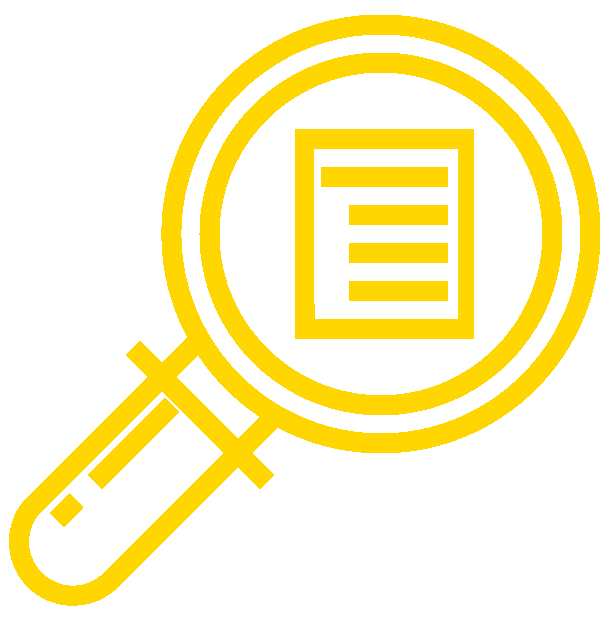## Learning OutcomesThe content, assignments, and assessments for Calculus II are aligned to the following learning outcomes. A full list of course learning outcomes can be viewed here: Calculus II Learning Outcomes.

## Module 1: Use basic integration techniques to calculate area

• Apply summation rules
• Interpret definite integrals
• Explain the Fundamental Theorem of Calculus
• Use the net change theorem
• Apply substitution to indefinite and definite integrals
• Integrate functions involving exponential and logarithmic functions
• Integrate functions resulting in inverse trigonometric functions
• Approximate integrals when the antiderivative is impossible to calculate

## Module 2: Apply integrals to geometric application, physical application, and modeling problems

• Calculate the areas of curved regions by using integration methods
• Find the volume of a solid of revolution using various methods
• Compare different integration methods for determining volume
• Calculate the arc length of a curve and the surface area of a solid of revolution
• Quantify mass, density, work, force, and pressure using integration
• Determine the center of mass in various dimensions
• Apply integration and derivatives to exponential and natural logarithmic functions
• Apply the exponential growth model to explain real world concepts
• Use integrals and derivatives to evaluate hyperbolic functions

## Module 3: Perform additional integration calculations and approximations

• Apply the integration-by-parts formula to solve indefinite and definite integrals
• Solve integration problems involving trigonometric functions
• Solve integration problems involving trigonometric substitution
• Identify linear and quadratic factors in rational functions
• Solve integration problems using alternative strategies
• Use numerical integration techniques to determine the accuracy of integrals
• Evaluate improper integrals

## Module 4: Develop methods to solve differential equations

• Analyze differential equations and their solutions
• Evaluate direction fields of first-order differential equations
• Apply separation of variables to differential equations
• Interpret the results and solution curves of logistic equations
• Solve first-order linear equations

## Module 5: Understand infinite series and how to use them to evaluate functions

• Evaluate sequences by determining the formula, the limit, and the divergence
• Interpret infinite, geometric, and telescoping series
• Use the divergence and integral tests to determine the convergence or divergence of a series
• Use the comparison test to determine the convergence of a series
• Assess alternating series by testing for convergence and estimating the sum
• Apply the ratio and root tests to a series

## Module 6: Represent functions using power series

• Use power series to represent functions and determine convergence
• Apply the properties of a power series
• Examine Taylor and Maclaurin series
• Apply Taylor series to solve differential equations and nonelementary integrals

## Module 7: Describing curves through parametric equations and polar coordinates

• Identify parametric equations
• Apply calculus to parametric equations
• Understand polar coordinates and their application
• Determine area and arc length in polar coordinates
• Distinguish properties of parabolas, ellipses, and hyperbolas